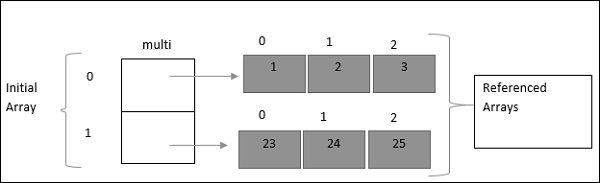# TypeScript - Multidimensional Arrays

An array element can reference another array for its value. Such arrays are called as multidimensional arrays. TypeScript supports the concept of multi-dimensional arrays. The simplest form of a multi-dimensional array is a two-dimensional array.

## Declaring a Two-Dimensional array

```var arr_name:datatype[][]=[ [val1,val2,val3],[v1,v2,v3] ]
```

## Accessing a Two-Dimensional array element

```var arr_name:datatype[initial_array_index][referenced_array_index] = [ [val1,val2,val 3],
[v1,v2,v3] ]
```

The following example better explains this concept.

## Example

```var multi:number[][] = [[1,2,3],[23,24,25]]
console.log(multi)
console.log(multi)
console.log(multi)
console.log(multi)
console.log(multi)
console.log(multi)
```

The above example initially declares an array with 2 elements. Each of these elements refer to another array having 3 elements. The pictorial representation of the above array is given below.While referring to an array element here, the subscript of the initial array element must be followed by the subscript of the referenced array element. This is illustrated in the code.

On compiling, it will generate following JavaScript code.

```//Generated by typescript 1.8.10
var multi = [[1, 2, 3], [23, 24, 25]];
console.log(multi);
console.log(multi);
console.log(multi);
console.log(multi);
console.log(multi);
console.log(multi);
```

The output of the above code is as follows −

```1
2
3
23
24
25
```
typescript_arrays.htm